RS Aggarwal Class 8 Solutions Chapter 11 - Compound Interest

RS Aggarwal Class 8 Chapter 11 - Compound Interest Solutions Free PDF

Compound Interest is the addition of interest to the principal sum. In this chapter, the students will learn how to calculate compound interest, principal, and interest rate. Learn the concepts of compound interest by solving questions from RS Aggarwal. This book has all the concepts explained in an easy and better way. To help students solve the questions from RS Aggarwal we have also provided the RS Aggarwal class 8 solutions chapter 11 compound interest.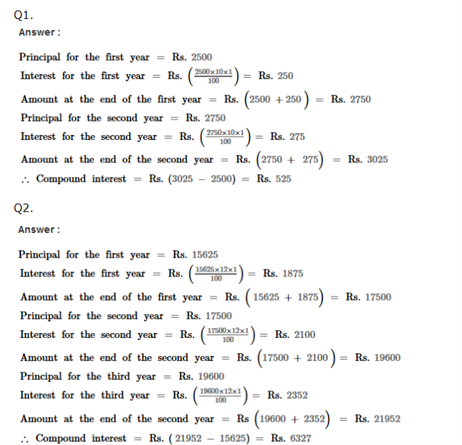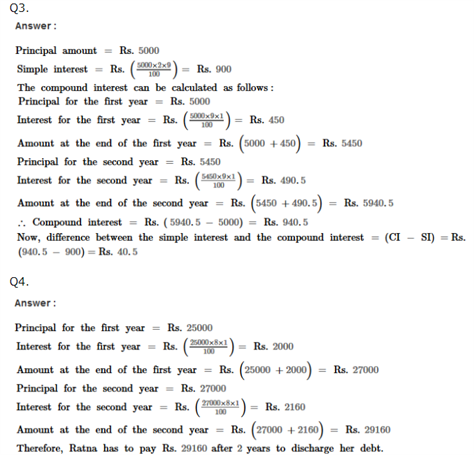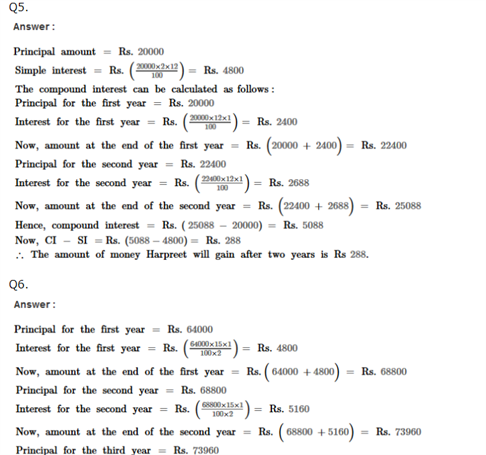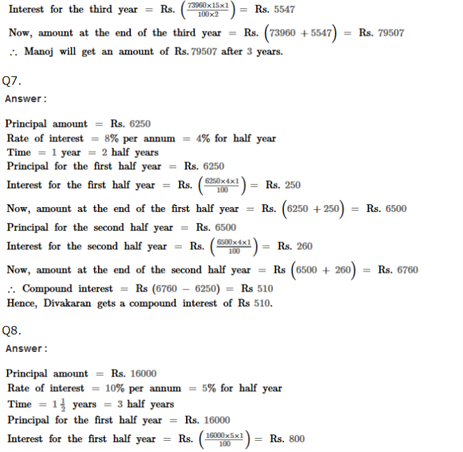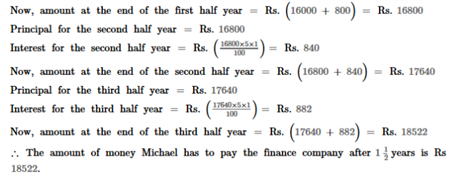Practise This Question

Which of the following is not a three-dimensional shape?